Courses

# Test: File System & Device Management- 2

## 15 Questions MCQ Test Question Bank for GATE Computer Science Engineering | Test: File System & Device Management- 2

Description
This mock test of Test: File System & Device Management- 2 for Computer Science Engineering (CSE) helps you for every Computer Science Engineering (CSE) entrance exam. This contains 15 Multiple Choice Questions for Computer Science Engineering (CSE) Test: File System & Device Management- 2 (mcq) to study with solutions a complete question bank. The solved questions answers in this Test: File System & Device Management- 2 quiz give you a good mix of easy questions and tough questions. Computer Science Engineering (CSE) students definitely take this Test: File System & Device Management- 2 exercise for a better result in the exam. You can find other Test: File System & Device Management- 2 extra questions, long questions & short questions for Computer Science Engineering (CSE) on EduRev as well by searching above.
QUESTION: 1

### Determine the number of page faults when references to pages occur in the order -1, 2,4, 5, 2,1,2, 4. Assume that the main memory can accommodate 3 pages and the main memory already has the pages 1 and 2, with page 1 having been brought earlier than page 2. (Assume LRU algorithm is used)

Solution:

1, 2, 4, 5, 2, 1 , 2 , 4
Since 1 and 2 are already in the memory which can accomodate 3 pages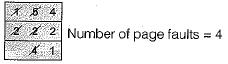QUESTION: 2

### Working set (t, k) at an instant of time t, is the set of

Solution:

Explanation : Working set (t, k) at an instant of time t, is the k set of pages that have been referenced in the last t time units.

QUESTION: 3

### Disk scheduling involves deciding

Solution:

Disk scheduling manages the disk access and order of request to be fulfilled. It does not have the bounds in determining the physical location, where actually the files are stored.

QUESTION: 4

In which of the following directory systems, is it possible to have multiple complete paths for a file, starting from the root directory?

Solution:

In Acyclic Graph directory file can be accessed using different paths from the same root directory this can be done with the helps of soft links.

QUESTION: 5

A process refers to 5 pages, A, B, C, D and E in the order - A, B, C, D, A, B, E, A, B, C, D, E. If the page replacement algorithm is FIFO, the number of pages which transfer with an empty internal store of 3 frames is

Solution:

The first 3 reference A, B, C fills the internal storage with A, B,C in 3 page transfers. Now the next reference D results in a page fault. So page A is downloaded and D takes its place after a page transfer. So, the internal store has D, Fand C. The next reference is A - results in a page fault. So, a page transfer takes place and swaps B and A. Continuing this way, we find totally 9 page transfers are necessary.

QUESTION: 6

In question number 25, if the number of available page frames is increased to 4 then the number of page transfer

Solution:

We find the required number of page transfer is 10. So, increasing the number of pages need not necessarily reduce the number of page faults. It is the actual sequences of references that decides.

QUESTION: 7

Disk requests come to a disk driver for cylinders in the order 10,22,20,2,40,6 and 38, at a time when the disk drive is reading from cylinder 20. The seek time is 6 ms per cylinder. The total seek time, if the disk arm scheduling algorithm is first-come-first-served is

Solution:

The disk drive has to traverse totally 146 cylinders (verify). So, seek time is 6 x 146 = 876 ms.

QUESTION: 8

In question 29, if the scheduling algorithm is the closest cylinder next, then the total seek time will be

Solution: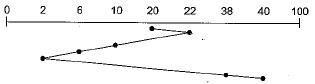Total seek time:
[2 + 12 + 4 + 4 + 36 + 2] x 6 msec = 360 msec

QUESTION: 9

A certain moving arm disk storage with one head has following specifications:
Number of tracks/recording surface = 200
Disk rotation speed = 2400 rpm
Track storage capacity = 62500 bits
The average latency time (assume that the head can move from one track to another only by traversing the entire track) is

Solution:

To cover 2400 x 62500 bits, 60 s are needed. Average latency time is the time needed to traverse 100 tracks i.e. 100 x 62500 bits, which is 2.5 s.

QUESTION: 10

The address sequence generated by tracing a particular program executing in a pure demand paging system with 100 records per page, with 1 free main memory frame is recorded as follows. What is the number of page faults?
0100, 0200, 0430, 0499, 0510, 0530, 0560, 0120, 0220, 0240, 0260, 0320, 0370

Solution:

When it tries to access 0100, it results in a page fault as the memory is empty right now. So, it loads the second page (which has the addresses 100 - 199). Trying to access 200 will result in a page fault, as it is not in memory right now. So the third page with the addresses from 200 to 299 will replace the second page in memory. Trying to access 430 will result in another page fault. Proceeding this way, we find trying to access the addresses 0510,0120,0220 and 0320 will all result in page faults. So, altogether 7 page faults.

QUESTION: 11

A memory page containing a heavily used variable that was initialized very early and is in constant use is removed, when the page replacement algorithm used is

Solution:

Since the page is used heavily then it can not be removed if LFU used, since it is in constant used, hence it can’t be removed if LRU is used. Using FIFO only it can be removed effectively.

QUESTION: 12

There are five virtual pages, numbered from 0 to 4. The page are referenced in the following order
0 1 2 3 0 1 4 0 1 2 3 4
If FIFO page replacement algorithm is used then find:
1. Number of page replacement faults that occur if 3 page frames are present.
2. Number of page faults that occur if 4 page frames are present.

Solution:

If 3 page frames are used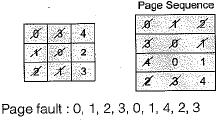Page hit : 0, 1, 4.
∴ Number of page fault = 9
If 4 pages frames are used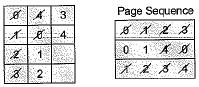Page faults ; 0, 1, 2, 3, 4, 0, 1,2, 3, 4 Page hit : 0, 1
∴ Number of page faults = 10

QUESTION: 13

Given references to the following pages by a program
0, 9, 0, 1,8, 1,8, 7, 8, 7, 1,2, 8, 2, 7, 8, 2, 3, 8,3 How many page faults will occur if the program has been three page frames available to it and uses an optimal replacement?

Solution:

Page sequence 0, 9, 0, 1,8, 1,8, 7, 8, 7, 1,2, 3, 2, 7, 8, 2, 3, 8, 3
Given 3 page frames are available and optimal replacement policy.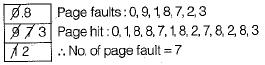QUESTION: 14

A virtual memory system uses First In First Out (FIFO) page replacement policy and allocates a fixed number of frames to a process. Consider the following statements:
P : Increasing the number of page frames allocated to a process sometimes increases the page fault rate.
Q : Some program do not exhibit locality of reference.Which one of the following is TRUE?

Solution:
• FIFO suffers from BELADY’s anomaly, in which sometimes page faults increase with increase in page frames. Hence, true.
• Locality of reference depends on demand of process to access consecutive data and hence locality of reference may not always be satisfied. Hence, true
QUESTION: 15

On a disk with 1000 cylinders, numbers 0 to 999, compute the number of tracks the disk arm must move to satisfy all the requests in the disk queue. Assume the last request serviced was at tracks 345 and the head is moving towards track 0. The queue in FIFO order contains requests for the following tracks. 123, 874, 692, 475, 105, 376. Perform the computation using C-SCAN scheduling algorithm. What is the total distance?

Solution: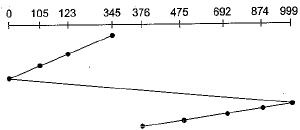∴ Total distance covered by the head
= (345 -123) + (123 -105) + (105 - 0) + (999 - 0)
+ (999-874) + (874-692) + (692-475) + (475 -376)
= 222 + 18 + 105 + 999 + 125 + 182 + 217 + 99
= 1967